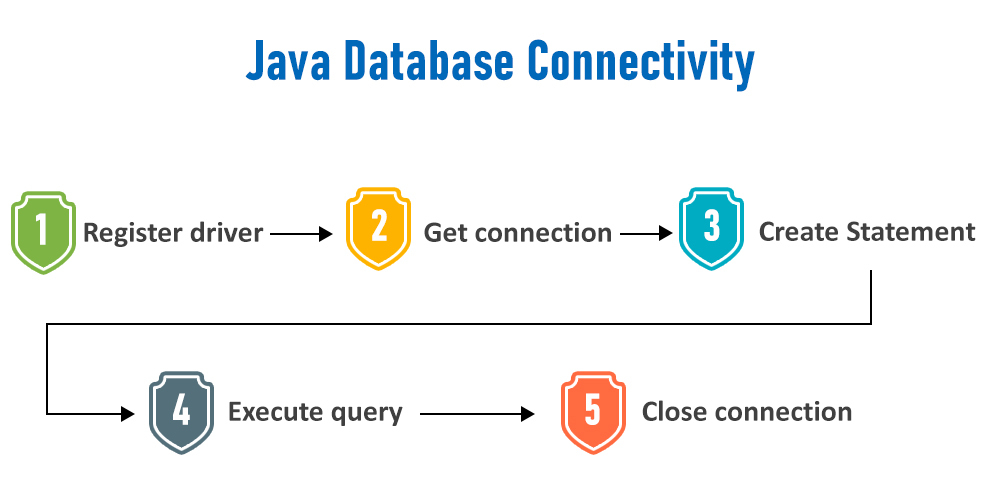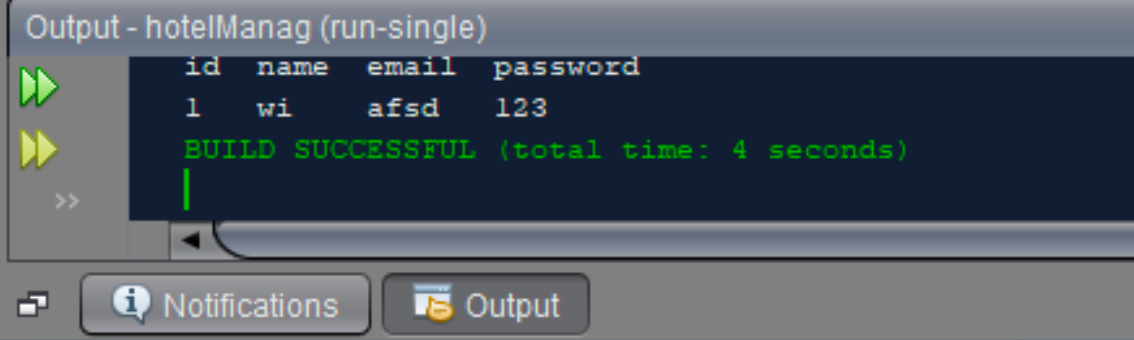# Java Program to Search the Contents of a Table in JDBC

• Last Updated : 27 Oct, 2021

In order to deal with JDBC standard 7 steps are supposed to be followed:

1. Import the database
3. Create a connection
4. Create a statement
5. Execute the query
6. Process the results
7. Close the connectionProcedure:

1. Import the database-syntax  for importing the  sql database in java is-
import java.sql.* ;
forName(com.mysql.jdbc.xyz) ;
3. Creating a database irrespective of SQL or NoSQL. Creating a database using sqlyog and creating some tables in it and fill data inside it in order to search for the contents of a table. For example, the database is named as “hotelman” and table names are “cuslogin” and “adminlogin”.
4. Create a connection: Open any IDE where the java executable file can be generated following the standard methods. Creating a package further creating the class. Inside the package, open a new java file and type the below code for JDBC connectivity and save the filename with connection.java.
5. Searching content in a table, let’s suppose my “cuslogin” table has columns namely “id”, “name”, “email”, “password” and we want to search the customer whose id is 1.
6. Initialize a string with the SQL query as follows
`String sql="select * from cuslogin where id=1";`

If we want to search for any id in general, then the SQL query becomes

`String sql="select * from cuslogin where id="+Integer.parseInt(textfield.getText());`

The textfield is the area(in Jframe form) where the user types the id he wants to search in the “cuslogin” table.

4.1: Initialize the below objects of Connection class, PreparedStatement class, and ResultSet class(needed for JDBC) and connect with the database as follows

```Connection con = null;
PreparedStatement p = null;
ResultSet rs = null;
con = connection.connectDB();```

4.2: Now, add the SQL query of step 3.1 inside prepareStatement and execute it as follows:

```p =con.prepareStatement(sql);
rs =p.executeQuery();```

4.3: We check if rs.next() is not null, then we display the details of that particular customer present in “cuslogin” table

4.4: Open a new java file (here, its result.java) inside the same package and type the full code (shown below) for searching the details of the customer whose id is 1, from table “cuslogin”.

Note: both the file viz result.java and connection.java should be inside the same package, else the program won’t give desired output!!

Implementation:

Example 1

Connection class of JDBC by making an object to be invoked in main(App) java program below in 1B

## Java

 `// Java program to search the contents of``// a table in JDBC Connection class for JDBC``// Connection class of JDBC` `// Importing required classes``import` `java.sql.Connection;``import` `java.sql.DriverManager;``import` `java.sql.SQLException;` `public` `class` `connectionDB {` `    ``final` `String DB_URL``        ``= ``"jdbc:mysql://localhost:3306/testDB?useSSL=false"``;` `    ``//  Database credentials` `    ``// We need two parameters to access the database``    ``// Root and password``    ` `    ``// 1. Root``    ``final` `String USER = ``"root"``;``    ` `    ``// 2. Password to fetch database``    ``final` `String PASS = ``"Imei@123"``;` `    ``// Connection class for our database connectivity``   ``public` `Connection connectDB()``    ``{``        ``// Initially setting NULL``        ``// to connection class object``        ``Connection con = ``null``;` `        ``// Try block to check exceptions``        ``try` `{` `            ``// Loading DB(SQL) drivers``            ``Class.forName(``"com.mysql.cj.jdbc.Driver"``);` `            ``// Registering SQL drivers``            ``con = DriverManager.getConnection(DB_URL, USER,``                                              ``PASS);``        ``}` `        ``// Catch block to handle database exceptions``        ``catch` `(SQLException e) {` `            ``// Print the line number where exception occurs``            ``e.printStackTrace();``        ``}` `        ``// Catch block to handle exception``        ``// if class not found``        ``catch` `(ClassNotFoundException e) {` `            ``// Function prints the line number``            ``// where exception occurs``            ``e.printStackTrace();``        ``}` `        ``// Returning Connection class object to``        ``// be used in (App/Main) GFG class``        ``return` `con;``    ``}``}`

App/Main Class where the program is compiled and run calling the above connection class object

## Java

 `// Java program to Search the``// contents of a table in JDBC` `// Main Java program (App Class) of JDBC` `// Step 1: Importing database files``// Importing SQL libraries``import` `java.sql.*;` `// Main class``// It's connection class is shown above``public` `class` `GFG {` `    ``// Main driver method``    ``public` `static` `void` `main(String[] args)``    ``{``        ``// Step 2: Establishing a connection``        ``connectionDB connection = ``new` `connectionDB();` `        ``// Assigning NULL to object of Connection class``        ``// as shown returned by above program``        ``Connection con = ``null``;``        ``PreparedStatement p = ``null``;``        ``ResultSet rs = ``null``;` `        ``// Step 3: Loading and registereding drivers``        ``// Loaded and registered in Connection class``        ``// shown in above program``        ``con = connection.connectDB();` `        ``// Try block to check exceptions``        ``try` `{` `            ``// Step 4: Write a statement``            ``String sql``                ``= ``"select * from cuslogin where id=1"``;` `            ``// Step 5: Execute the query``            ``p = con.prepareStatement(sql);``            ``rs = p.executeQuery();` `            ``// Step 6: Process the results``            ``System.out.println(``                ``"id\t\tname\t\temail\t\tpassword"``);` `            ``// Condition check using next() method``            ``// Holds true till there is single element remaining``          ``// in the object``            ``if` `(rs.next()) {``              ` `                ``int` `id = rs.getInt(``"id"``);``                ``String name = rs.getString(``"name"``);``                ``String email = rs.getString(``"email"``);``                ``String password = rs.getString(``"password"``);``                ` `              ``// Print and display name, emailID and password``              ``System.out.println(id + ``"\t\t"` `+ name``                                   ``+ ``"\t\t"` `+ email + ``"\t\t"``                                   ``+ password);``            ``}``        ``}` `        ``// Catch block to handle exceptions``        ``catch` `(SQLException e) {` `            ``// Print the exception``            ``System.out.println(e);``        ``}``    ``}``}`

Output: Based on the values stored inside the “cuslogin” table.My Personal Notes arrow_drop_up